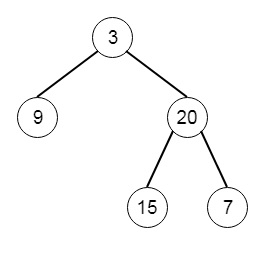# Construct Binary Tree from Preorder and Inorder Traversal in Python

Suppose we have the inorder and preorder traversal sequence of a binary tree. We have to generate the tree from these sequences. So if the preorder and inorder sequences are [3,9,20,15,7] and [9,3,15,20,7], then the tree will be −Let us see the steps −

• Suppose the method is called buildTree with preorder and inorder lists
• root := first node from the preorder, and delete first node from preorder
• root_index := index of root.val from the inorder list
• left or root := buildTree(preorder, subset of inorder from 0 to root_index)
• right or root := buildTree(preorder, subset of inorder from root_index + 1 to end)

Let us see the following implementation to get better understanding −

## Example

Live Demo

class TreeNode:
def __init__(self, data, left = None, right = None):
self.data = data
self.left = left
self.right = right
def print_tree(root):
#print using inorder traversal
if root is not None:
print_tree(root.left)
print(root.data, end = ',')
print_tree(root.right)
class Solution(object):
def buildTree(self, preorder, inorder):
if inorder:
root = TreeNode(preorder.pop(0))
root_index = inorder.index(root.data)
root.left = self.buildTree(preorder,inorder[:root_index])
root.right = self.buildTree(preorder,inorder[root_index+1:])
return root
ob1 = Solution()
print_tree(ob1.buildTree([3,9,20,15,7], [9,3,15,20,7]))

## Input

[3,9,20,15,7]
[9,3,15,20,7]

## Output

9, 3, 15, 20, 7,